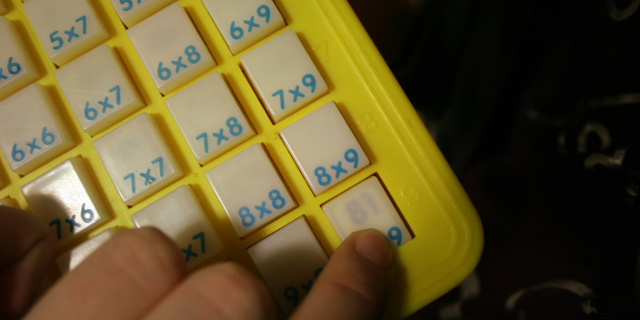# Unit cost vs. bit cost in time complexity

yourbasic.orgUnit-cost multiplication

Unit cost and bit cost are two different cost functions used to compute space and time complexity.

• Unit cost is used in a simplified model where a number, of any size, fits within a memory cell, and where standard arithmetic operations take constant time.
• With bit cost we take into account that computations with bigger numbers can be more expensive.

Unit cost often works well in practice as modern processors can perform arithmetics on 64-bit integer and floating point numbers in constant time.

## Unit cost example

``````func Add(x, y int64) int64 {
return x + y
}
``````

In this simple example it’s sensible to use unit cost and say that the function runs in constant time.

## Bit cost example

``````func Concatenate(x, y string) string {
return x + y
}
``````

Here it would be a mistake to use unit cost for the `+` operation: to concatenate two strings, we must allocate new memory and copy the characters.

In this case it’s better to use bit cost for the `+` operation:

• the length of the strings is used to measure the input size,
• and we say that the function runs in Θ(len(x) + len(y)) time.
Note that we still use unit cost for character operations. This makes perfect sense as characters can be represented by small fixed-sized integers.